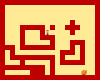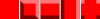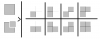# 2D Ambient Occlusion Using Tiles

#### Zerb Games

##### Member
I've been trying to make 2D Ambient Occlusion to spice up my game a bit. So far I've gone with a few techniques, first shaders, then surfaces, and now I want to try tiles. However, I'm way over my head, It takes 256 individual tiles to make the entire map. I saw while reading the Documentation that there is a tile xscale, and yscale, for flipping and stuffs. Which would cut down on the amount of tiles needed, but the logic is very heavy. So far I'm pretty much lost, the concept is there, but I don't really know how to execute it.

Anybody mind whipping up a script for me, or helping me understand how to do this. I'm not the best person when it comes to logic like this. Thank you!Here's what I got so far:#### dphsw

##### Member
It seems like you're doing something similar to what I was doing yesterday. (Actually I was just adding shading to my tiles, but the concept is more or less the same.) It's subtle, but there's a few pixels of lighter shading on the top and left of all the tiles, and a few pixels of darker shading on the bottom and right of them.I did once make a game where I made all these tiles individually. I assume you're getting the figure of 256 tiles because there's 4 edges and 4 corners, so 8 possible bits that could be shaded or not, and 2^8=256. But actually not all of those can happen - you'll never get an edge shaded without the two corners next to it shaded, and stuff like that cuts down the number of possibilities. But anyway, it's still a lot if you draw them all individually. 47, I believe.

As it is, I actually only drew 5 tiles. The 5 tiles looked like this (drawn a bit less subtly for emphasis):Then instead of drawing a whole tile at a time, I only draw a quarter of the tile at a time using the draw_sprite_part function, but check the four tiles around it to see which quarter is appropriate. If both the bit to the side, the bit above/below (depending on whether it's an upper or lower quarter) and the bit at the corner are taken up with tiles, I draw a quarter of the first tile. If none of them are taken up, I draw a quarter of the second tile. If the bit above/below and to the side are taken up, but not the corner, I draw a quarter of the fifth tile. And so on. It means you don't have to draw, for example, a tile with a U shape (in four orientations) - that will just happen by drawing the second tile for two of the quarters, and the third or fourth tile for the other two.
It has lowered my average framerate, which I expected, but I have always intended later on to move to drawing the level tiles and background to a separate surface when the level starts anyway, so I'm not concerned about that.

Anyway, I think the same process of splitting things into corners, instead of doing a whole tile at a time, could work for you. If you're doing the lighting evenly, you can even use the same shading for top, bottom, left and right, but I'd recommend keeping them separate like I have done - you may at some point want to make the light look like it's coming from a diagonal angle, so that there's more shading on one side than there is on another.

Here is my code for doing the shading:
Code:
``````/*
// layout of sprites in the spritestrip
0-plain
1-all edges
2-horizontal edges
3-vertical edges
4-corners
5-platform plain
6-platform edges

// overlay_solid is initialised in create event
// tells us what sprite is appropriate depending on the 'bits' variable
overlay_solid = 0;
overlay_solid = 3;
overlay_solid = 2;
overlay_solid = 1;
overlay_solid = 4;
overlay_solid = 3;
overlay_solid = 2;
overlay_solid = 1;

*/

var i,j,t;
// Set these variables to the appropriate spritestrips for what world we are in
var background = bck_world0;
var level = spr_tiles_world_0;

// tiles around
var row, col, corner, bits;

for(j=0;j<LEVEL_HEIGHT;j++){
for(i=0;i<LEVEL_WIDTH;i++){

// Draw Background
t = lf_grid_background[# i,j];
draw_sprite(background,t,i*TILE_SIZE,j*TILE_SIZE);

// Draw Level Tile
t = lf_grid_level_tiles[# i,j];

if(t==TILE_SOLID){
draw_sprite(level,0,i*TILE_SIZE,j*TILE_SIZE);
if(i>0){
row = lf_grid_level_tiles[# i-1,j];
if(j>0){
col = lf_grid_level_tiles[# i,j-1];
corner = lf_grid_level_tiles[# i-1,j-1];
bits = 0;
if(row==TILE_SOLID) bits++;
if(col==TILE_SOLID) bits+=2;
if(corner==TILE_SOLID) bits+=4;
draw_sprite_part(level,overlay_solid[bits],0,0,HALF_TILE_SIZE,HALF_TILE_SIZE,i*TILE_SIZE,j*TILE_SIZE);
}
if(j<LEVEL_HEIGHT-1){
col = lf_grid_level_tiles[# i,j+1];
corner = lf_grid_level_tiles[# i-1,j+1];
bits = 0;
if(row==TILE_SOLID) bits++;
if(col==TILE_SOLID) bits+=2;
if(corner==TILE_SOLID) bits+=4;
draw_sprite_part(level,overlay_solid[bits],0,HALF_TILE_SIZE,HALF_TILE_SIZE,HALF_TILE_SIZE,i*TILE_SIZE,j*TILE_SIZE + HALF_TILE_SIZE);
}
}
if(i<LEVEL_WIDTH-1){
row = lf_grid_level_tiles[# i+1,j];
if(j>0){
col = lf_grid_level_tiles[# i,j-1];
corner = lf_grid_level_tiles[# i+1,j-1];
bits = 0;
if(row==TILE_SOLID) bits++;
if(col==TILE_SOLID) bits+=2;
if(corner==TILE_SOLID) bits+=4;
draw_sprite_part(level,overlay_solid[bits],HALF_TILE_SIZE,0,HALF_TILE_SIZE,HALF_TILE_SIZE,i*TILE_SIZE + HALF_TILE_SIZE,j*TILE_SIZE);
}
if(j<LEVEL_HEIGHT-1){
col = lf_grid_level_tiles[# i,j+1];
corner = lf_grid_level_tiles[# i+1,j+1];
bits = 0;
if(row==TILE_SOLID) bits++;
if(col==TILE_SOLID) bits+=2;
if(corner==TILE_SOLID) bits+=4;
draw_sprite_part(level,overlay_solid[bits],HALF_TILE_SIZE,HALF_TILE_SIZE,HALF_TILE_SIZE,HALF_TILE_SIZE,i*TILE_SIZE + HALF_TILE_SIZE,j*TILE_SIZE + HALF_TILE_SIZE);
}
}
}

}
}``````

Last edited:

#### NightFrost

##### Member
Why didn't a shader suit you? Going by the image you posted, a heavy gaussian blur shader applied to a black copy of the tiles would be what you want.

R

#### renex

##### Guest
•Zerb Games

#### NightFrost

##### Member
Oh, I'll need to test that corner method at some point. I tend to use the method described here, but this sounds nice by the virtue of having to spend less time on creating tilesets.

•Zerb Games

#### Zerb Games

##### Member
Slow.

Yup!
View attachment 3610
By processing only a corner at a time you cut all possible tile combinations down to fewer tiles, and then it becomes a very simple process.
I would like to know more.

It seems like you're doing something similar to what I was doing yesterday. (Actually I was just adding shading to my tiles, but the concept is more or less the same.) It's subtle, but there's a few pixels of lighter shading on the top and left of all the tiles, and a few pixels of darker shading on the bottom and right of them.

View attachment 3603

I did once make a game where I made all these tiles individually. I assume you're getting the figure of 256 tiles because there's 4 edges and 4 corners, so 8 possible bits that could be shaded or not, and 2^8=256. But actually not all of those can happen - you'll never get an edge shaded without the two corners next to it shaded, and stuff like that cuts down the number of possibilities. But anyway, it's still a lot if you draw them all individually. 47, I believe.

As it is, I actually only drew 5 tiles. The 5 tiles looked like this (drawn a bit less subtly for emphasis):
View attachment 3605
Then instead of drawing a whole tile at a time, I only draw a quarter of the tile at a time using the draw_sprite_part function, but check the four tiles around it to see which quarter is appropriate. If both the bit to the side, the bit above/below (depending on whether it's an upper or lower quarter) and the bit at the corner are taken up with tiles, I draw a quarter of the first tile. If none of them are taken up, I draw a quarter of the second tile. If the bit above/below and to the side are taken up, but not the corner, I draw a quarter of the fifth tile. And so on. It means you don't have to draw, for example, a tile with a U shape (in four orientations) - that will just happen by drawing the second tile for two of the quarters, and the third or fourth tile for the other two.
It has lowered my average framerate, which I expected, but I have always intended later on to move to drawing the level tiles and background to a separate surface when the level starts anyway, so I'm not concerned about that.

Anyway, I think the same process of splitting things into corners, instead of doing a whole tile at a time, could work for you. If you're doing the lighting evenly, you can even use the same shading for top, bottom, left and right, but I'd recommend keeping them separate like I have done - you may at some point want to make the light look like it's coming from a diagonal angle, so that there's more shading on one side than there is on another.

Here is my code for doing the shading:
Code:
``````/*
// layout of sprites in the spritestrip
0-plain
1-all edges
2-horizontal edges
3-vertical edges
4-corners
5-platform plain
6-platform edges

// overlay_solid is initialised in create event
// tells us what sprite is appropriate depending on the 'bits' variable
overlay_solid = 0;
overlay_solid = 3;
overlay_solid = 2;
overlay_solid = 1;
overlay_solid = 4;
overlay_solid = 3;
overlay_solid = 2;
overlay_solid = 1;

*/

var i,j,t;
// Set these variables to the appropriate spritestrips for what world we are in
var background = bck_world0;
var level = spr_tiles_world_0;

// tiles around
var row, col, corner, bits;

for(j=0;j<LEVEL_HEIGHT;j++){
for(i=0;i<LEVEL_WIDTH;i++){

// Draw Background
t = lf_grid_background[# i,j];
draw_sprite(background,t,i*TILE_SIZE,j*TILE_SIZE);

// Draw Level Tile
t = lf_grid_level_tiles[# i,j];

if(t==TILE_SOLID){
draw_sprite(level,0,i*TILE_SIZE,j*TILE_SIZE);
if(i>0){
row = lf_grid_level_tiles[# i-1,j];
if(j>0){
col = lf_grid_level_tiles[# i,j-1];
corner = lf_grid_level_tiles[# i-1,j-1];
bits = 0;
if(row==TILE_SOLID) bits++;
if(col==TILE_SOLID) bits+=2;
if(corner==TILE_SOLID) bits+=4;
draw_sprite_part(level,overlay_solid[bits],0,0,HALF_TILE_SIZE,HALF_TILE_SIZE,i*TILE_SIZE,j*TILE_SIZE);
}
if(j<LEVEL_HEIGHT-1){
col = lf_grid_level_tiles[# i,j+1];
corner = lf_grid_level_tiles[# i-1,j+1];
bits = 0;
if(row==TILE_SOLID) bits++;
if(col==TILE_SOLID) bits+=2;
if(corner==TILE_SOLID) bits+=4;
draw_sprite_part(level,overlay_solid[bits],0,HALF_TILE_SIZE,HALF_TILE_SIZE,HALF_TILE_SIZE,i*TILE_SIZE,j*TILE_SIZE + HALF_TILE_SIZE);
}
}
if(i<LEVEL_WIDTH-1){
row = lf_grid_level_tiles[# i+1,j];
if(j>0){
col = lf_grid_level_tiles[# i,j-1];
corner = lf_grid_level_tiles[# i+1,j-1];
bits = 0;
if(row==TILE_SOLID) bits++;
if(col==TILE_SOLID) bits+=2;
if(corner==TILE_SOLID) bits+=4;
draw_sprite_part(level,overlay_solid[bits],HALF_TILE_SIZE,0,HALF_TILE_SIZE,HALF_TILE_SIZE,i*TILE_SIZE + HALF_TILE_SIZE,j*TILE_SIZE);
}
if(j<LEVEL_HEIGHT-1){
col = lf_grid_level_tiles[# i,j+1];
corner = lf_grid_level_tiles[# i+1,j+1];
bits = 0;
if(row==TILE_SOLID) bits++;
if(col==TILE_SOLID) bits+=2;
if(corner==TILE_SOLID) bits+=4;
draw_sprite_part(level,overlay_solid[bits],HALF_TILE_SIZE,HALF_TILE_SIZE,HALF_TILE_SIZE,HALF_TILE_SIZE,i*TILE_SIZE + HALF_TILE_SIZE,j*TILE_SIZE + HALF_TILE_SIZE);
}
}
}

}
}``````
Wow! I appreciate the code sir, will have to check it outChanged the draw_sprite_part with tile add, so at the beginning of the level, all the lighting is done. Tiles are probably much more efficient.

Last edited:
P

#### Pup

##### Guest
It seems like you're doing something similar to what I was doing yesterday. (Actually I was just adding shading to my tiles, but the concept is more or less the same.) It's subtle, but there's a few pixels of lighter shading on the top and left of all the tiles, and a few pixels of darker shading on the bottom and right of them.

View attachment 3603

I did once make a game where I made all these tiles individually. I assume you're getting the figure of 256 tiles because there's 4 edges and 4 corners, so 8 possible bits that could be shaded or not, and 2^8=256. But actually not all of those can happen - you'll never get an edge shaded without the two corners next to it shaded, and stuff like that cuts down the number of possibilities. But anyway, it's still a lot if you draw them all individually. 47, I believe.

As it is, I actually only drew 5 tiles. The 5 tiles looked like this (drawn a bit less subtly for emphasis):
View attachment 3605
Then instead of drawing a whole tile at a time, I only draw a quarter of the tile at a time using the draw_sprite_part function, but check the four tiles around it to see which quarter is appropriate. If both the bit to the side, the bit above/below (depending on whether it's an upper or lower quarter) and the bit at the corner are taken up with tiles, I draw a quarter of the first tile. If none of them are taken up, I draw a quarter of the second tile. If the bit above/below and to the side are taken up, but not the corner, I draw a quarter of the fifth tile. And so on. It means you don't have to draw, for example, a tile with a U shape (in four orientations) - that will just happen by drawing the second tile for two of the quarters, and the third or fourth tile for the other two.
It has lowered my average framerate, which I expected, but I have always intended later on to move to drawing the level tiles and background to a separate surface when the level starts anyway, so I'm not concerned about that.

Anyway, I think the same process of splitting things into corners, instead of doing a whole tile at a time, could work for you. If you're doing the lighting evenly, you can even use the same shading for top, bottom, left and right, but I'd recommend keeping them separate like I have done - you may at some point want to make the light look like it's coming from a diagonal angle, so that there's more shading on one side than there is on another.

Here is my code for doing the shading:
Code:
``````/*
// layout of sprites in the spritestrip
0-plain
1-all edges
2-horizontal edges
3-vertical edges
4-corners
5-platform plain
6-platform edges

// overlay_solid is initialised in create event
// tells us what sprite is appropriate depending on the 'bits' variable
overlay_solid = 0;
overlay_solid = 3;
overlay_solid = 2;
overlay_solid = 1;
overlay_solid = 4;
overlay_solid = 3;
overlay_solid = 2;
overlay_solid = 1;

*/

var i,j,t;
// Set these variables to the appropriate spritestrips for what world we are in
var background = bck_world0;
var level = spr_tiles_world_0;

// tiles around
var row, col, corner, bits;

for(j=0;j<LEVEL_HEIGHT;j++){
for(i=0;i<LEVEL_WIDTH;i++){

// Draw Background
t = lf_grid_background[# i,j];
draw_sprite(background,t,i*TILE_SIZE,j*TILE_SIZE);

// Draw Level Tile
t = lf_grid_level_tiles[# i,j];

if(t==TILE_SOLID){
draw_sprite(level,0,i*TILE_SIZE,j*TILE_SIZE);
if(i>0){
row = lf_grid_level_tiles[# i-1,j];
if(j>0){
col = lf_grid_level_tiles[# i,j-1];
corner = lf_grid_level_tiles[# i-1,j-1];
bits = 0;
if(row==TILE_SOLID) bits++;
if(col==TILE_SOLID) bits+=2;
if(corner==TILE_SOLID) bits+=4;
draw_sprite_part(level,overlay_solid[bits],0,0,HALF_TILE_SIZE,HALF_TILE_SIZE,i*TILE_SIZE,j*TILE_SIZE);
}
if(j<LEVEL_HEIGHT-1){
col = lf_grid_level_tiles[# i,j+1];
corner = lf_grid_level_tiles[# i-1,j+1];
bits = 0;
if(row==TILE_SOLID) bits++;
if(col==TILE_SOLID) bits+=2;
if(corner==TILE_SOLID) bits+=4;
draw_sprite_part(level,overlay_solid[bits],0,HALF_TILE_SIZE,HALF_TILE_SIZE,HALF_TILE_SIZE,i*TILE_SIZE,j*TILE_SIZE + HALF_TILE_SIZE);
}
}
if(i<LEVEL_WIDTH-1){
row = lf_grid_level_tiles[# i+1,j];
if(j>0){
col = lf_grid_level_tiles[# i,j-1];
corner = lf_grid_level_tiles[# i+1,j-1];
bits = 0;
if(row==TILE_SOLID) bits++;
if(col==TILE_SOLID) bits+=2;
if(corner==TILE_SOLID) bits+=4;
draw_sprite_part(level,overlay_solid[bits],HALF_TILE_SIZE,0,HALF_TILE_SIZE,HALF_TILE_SIZE,i*TILE_SIZE + HALF_TILE_SIZE,j*TILE_SIZE);
}
if(j<LEVEL_HEIGHT-1){
col = lf_grid_level_tiles[# i,j+1];
corner = lf_grid_level_tiles[# i+1,j+1];
bits = 0;
if(row==TILE_SOLID) bits++;
if(col==TILE_SOLID) bits+=2;
if(corner==TILE_SOLID) bits+=4;
draw_sprite_part(level,overlay_solid[bits],HALF_TILE_SIZE,HALF_TILE_SIZE,HALF_TILE_SIZE,HALF_TILE_SIZE,i*TILE_SIZE + HALF_TILE_SIZE,j*TILE_SIZE + HALF_TILE_SIZE);
}
}
}

}
}``````
I'm not sure I'm following the variables here, I mean I get HALF_TILE_SIZE, but what is lf_grid_level_tiles[# i,j];

R

#### renex

##### Guest
I would like to know more.
Each corner can have eight diferent arrangements. Here's a diagram:This is much less information than if you combined these corners into a single tile, because each tile can have 8 neighbors (making for a total of 2^8 = 256 arrangements) while a corner having only 3 neighbors creates only 2^3 = 8 variations. This way, you can have only 8 tiles and combine four with rotation to create a full tile.

#### dphsw

##### Member
I'm not sure I'm following the variables here, I mean I get HALF_TILE_SIZE, but what is lf_grid_level_tiles[# i,j];
That's because I'm using a ds_grid to store where there are tiles and where there aren't. 'lf' stands for 'level file' (I have some other 'lf_' grids that store stuff like player and enemy starting positions in case the level gets restarted), it basically just has two values right now, TILE_SOLID or TILE_EMPTY, which are macros indicating whether there's a tile in that square of the grid or not. I also use this ds_grid elsewhere for collisions with the level - if I want to know whether there's a collision at, say, (124,63), I just divide those coordinate by the tile size, (which is 16 here, so I get (7,3), rounding down ) and check whether that bit of the grid contains a solid tile. I haven't checked, but I suspect it's more efficient than using instances for level collision.

If you haven't seen the # notation before, it's basically just a quick way of looking up values in a ds_grid. It's documented here:
•